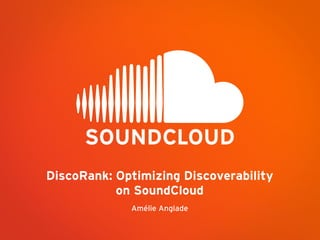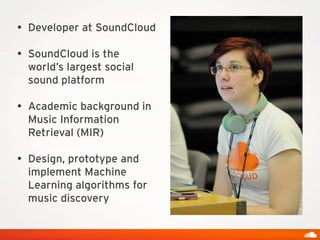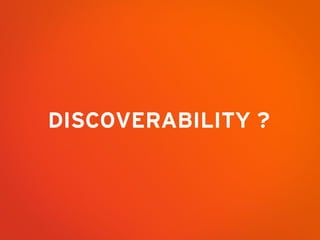Successfully reported this slideshow.

# DiscoRank: optimizing discoverability on SoundCloud×

# DiscoRank: optimizing discoverability on SoundCloud

These are the slides of the presentation I gave at the Realtime Conf EU on 23rd April 2013.
The full abstract of the talk can be found here: http://lanyrd.com/2013/realtime-conf-europe/scdtyf/

These are the slides of the presentation I gave at the Realtime Conf EU on 23rd April 2013.
The full abstract of the talk can be found here: http://lanyrd.com/2013/realtime-conf-europe/scdtyf/

### DiscoRank: optimizing discoverability on SoundCloud

1. 1. DiscoRank: Optimizing Discoverability on SoundCloud Amélie Anglade
2. 2. • Developer at SoundCloud • SoundCloud is the world’s largest social sound platform • Academic background in Music Information Retrieval (MIR) • Design, prototype and implement Machine Learning algorithms for music discovery
3. 3. DISCOVERABILITY ?
4. 4. PAGERANK
5. 5. • The web is a graph: • nodes = web pages • edges = hyperlinks • The (Page)rank of a node depends on the link structure of the graph WEB AND PAGERANK
6. 6. RANDOM SURFER
7. 7. RANDOM SURFER A B C D 1/3 1/3 1/3
8. 8. RANDOM SURFER A B C D 1/3 1/3 1/3
9. 9. Nodes visited more often: • Nodes with many links • Coming from frequently visited nodes RANDOM SURFER A B C D E
10. 10. Adjacency matrix A COMPUTING THE PAGERANK A B C D E Transition probability matrix M Probability distribution of surfer’s position
11. 11. Adjacency matrix A COMPUTING THE PAGERANK A B C D E Transition probability matrix M Probability distribution of surfer’s position
12. 12. Adjacency matrix A COMPUTING THE PAGERANK A B C D E Transition probability matrix M Probability distribution of surfer’s position
13. 13. Adjacency matrix A COMPUTING THE PAGERANK A B C D E Transition probability matrix M Probability distribution of surfer’s position
14. 14. Adjacency matrix A COMPUTING THE PAGERANK A B C D E Transition probability matrix M Probability distribution of surfer’s position
15. 15. Adjacency matrix A COMPUTING THE PAGERANK A B C D E Transition probability matrix M Probability distribution of surfer’s position
16. 16. TELEPORT A B C D E
17. 17. TELEPORT A B C D E
18. 18. TELEPORT A B C D E
19. 19. If N nodes in graph, probability to teleport to any other node (including self) = 1/N TELEPORT A B C D E 1/N 1/N 1/N 1/N 1/N
20. 20. TELEPORT A B C D E 1/N 1/N 1/N 1/N α ? 1-α 1/N At regular node: invoke teleport operation with probability α and standard random walk with probability (1 - α)
21. 21. Probability distribution of the surfer at any time is a vector. COMPUTING THE PAGERANK That vector converges to a steady state: the PageRank vector.
22. 22. PAGERANK EQUATION
23. 23. SOUNDCLOUD DISCORANK
24. 24. DISCORANK A B C D EUser User Track Playlist favorite follow featured in
25. 25. • Search across People, Sounds, Sets, Groups • One unique rank vector that contains all entities • Weight the links based on the type of event: • User favorites Track • Track is featured in Playlist ... • New big (but sparse) adjacency matrix: UNIVERSAL SEARCH
26. 26. • How do we identify content that is trending? • The more recent a listen, favorite, etc. (event) the higher the weight • Multiply each event (=edge) by a time decay: • New adjacency matrix: BACK TO EXPLORE
27. 27. PERFORMANCE OPTIMIZATION
28. 28. • Millions of entities(=nodes) and events(=edges) • First DiscoRank: several hours of computation • Trimmed down to a few minutes using: • Sparse matrix • Optimized storage of the graph in memory • Versioned copies of the DiscoRank • So technically we could compute the DiscoRank realtime A VERY LARGE GRAPH
29. 29. • • Re-mapping entity ids • Memory optimization so the graph holds in memory: • All edges details are stored in memory in a byte[] • buffer the byte[] into an opaque byte block pool • no object • sort the buffered byte[] in place • On disk and when computing the DiscoRank: • Delta encoded ordered adjacency lists: • One “from” node, several “to” nodes • Delta encode the “to” node ids USING SPARSITY
30. 30. • We keep versioned copies of: • the DiscoRank vector of results • the DiscoRank graph • We rebuild the entire DiscoRank graph from scratch once a week • In between: • we create additional graph segments with new entities and events • and use as prior for the DiscoRank computation the results of the previous DiscoRank run • Side effect: • Also allows for experimentation VERSIONED DISCORANK
31. 31. • MySQL batch jobs • DiscoRank results stored in HDFS • At the end of every DiscoRank run we re-load it in ElasticSearch: • For each item we combine its Lucene score with its DiscoRank INTEGRATION IN OUR INFRASTRUCTURE
32. 32. Amélie Anglade Sound/Music Information Retrieval Engineer about.me/utstikkar @utstikkar We’re hiring! www.soundcloud.com